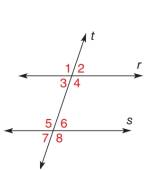Chapter 2.1, Problem 11E### Elementary Geometry for College St...

6th Edition
Daniel C. Alexander + 1 other
ISBN: 9781285195698

#### Solutions

Chapter
Section### Elementary Geometry for College St...

6th Edition
Daniel C. Alexander + 1 other
ISBN: 9781285195698
Textbook Problem
3 views

# Lines r and s are cut by the transversal t . Which angle a) corresponds to ∠ 1 ? b) is the alternate interior ∠ for ∠ 4 ? c) is the alternate exterior ∠ for ∠ 1 ? d) is the other interior angle on the same side of transversal t as ∠ 3 ?To determine

a)

To find:

The angle corresponds to the given angle.

Explanation

Given:

The given figure is,

r is parallel to s cut by a transversal t.

The given angle is 1.

Corresponding angles:

Corresponding angles are created where a transversal intersects two parallel lines.

The corresponding angles are the ones at the same location at each intersection. If the two lines are parallel, the corresponding angles are equal.

Approach:

Consider the given figure,

To determine

b)

To find:

The alternate interior angle for the given angle.

To determine

c)

To find:

The alternate exterior angle for the given angle.

To determine

d)

To find:

The other interior angle on the same side of transversal t for the given angle.

### Still sussing out bartleby?

Check out a sample textbook solution.

See a sample solution

#### The Solution to Your Study Problems

Bartleby provides explanations to thousands of textbook problems written by our experts, many with advanced degrees!

Get Started